## 加工现有 World Generator

Minecraft 原版的世界生成分为两个阶段，Generation 和 Population。我们将会对 Population 阶段进行修改：

``````public class ExtendVanillaGenerator implements Listener {

@EventHandler
public void onInit(WorldInitEvent event) {
if ("world".equals(event.getWorld().getName())) {
}
}

private static class PumpkinPopulator extends BlockPopulator {

@Override
public void populate(World world, Random random, Chunk chunk) {
// 随机生成一些南瓜的数量
int amount = random.nextInt(8);
for (int i = 0; i < amount; i++) {
// 随机位置
int x = random.nextInt(16);
int z = random.nextInt(16);
for (int y = 255; y >= 0; y--) {
if (chunk.getBlock(x, y, z).getType() != Material.AIR) {
// 只让南瓜生成在草方块上
if (chunk.getBlock(x, y, z).getType() == Material.GRASS_BLOCK
&& chunk.getBlock(x, y + 1, z).getType() == Material.AIR)
chunk.getBlock(x, y + 1, z).setType(Material.PUMPKIN);
break;
}
}
}
}
}
}``````Minecraft 原版的所有世界生成的类都在 nms 包内以 WorldGen 开头，可以自行反编译查看他们的实现：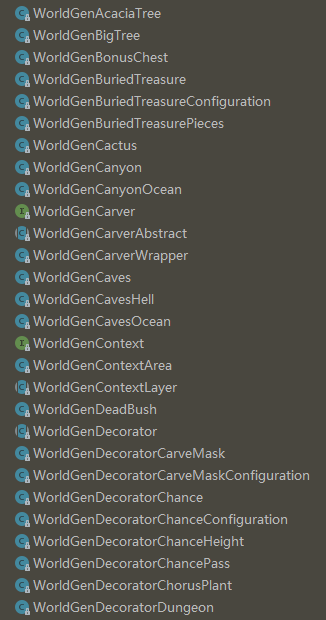## 简单的超平坦生成器

``````public class WorldGenTutor extends JavaPlugin {

@Override
public ChunkGenerator getDefaultWorldGenerator(String worldName, String id) {
return null;
}
}``````

``````worlds:
world:
generator: 插件名``````

### 实现一个 ChunkGenerator

``````public class FlatGenerator extends ChunkGenerator {

}``````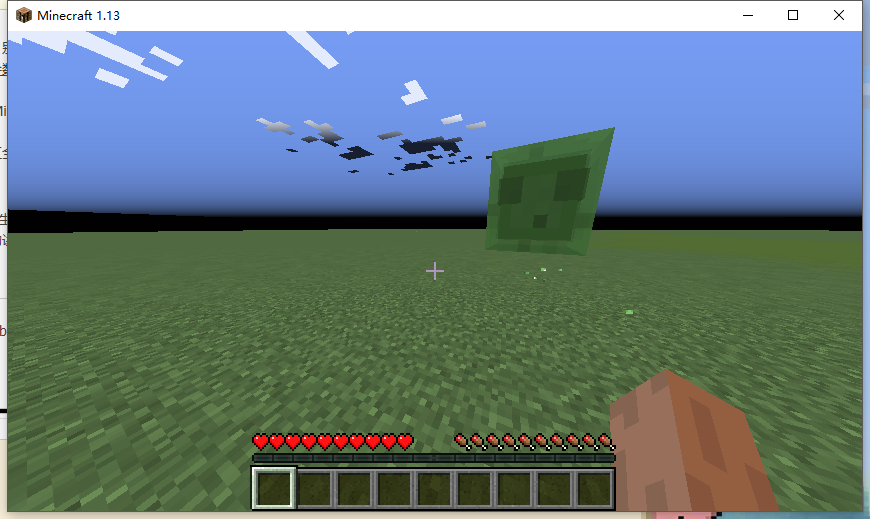``````public class FlatGenerator extends ChunkGenerator {

@Override
public ChunkData generateChunkData(World world, Random random, int x, int z, BiomeGrid biome) {
// 创建区块数据
ChunkData chunkData = createChunkData(world);
// 一个区块的大小为 16*16，高度为 0-255
// 将这个区块的 (0,0,0) 到 (16,2,16) ，即最低两层填充为基岩
chunkData.setRegion(0, 0, 0, 16, 2, 16, Material.BEDROCK);
// 将第三层填充为草方块
chunkData.setRegion(0, 2, 0, 16, 3, 16, Material.GRASS_BLOCK);
// 将整个区块的生物群系设置为平原（PLAINS）
for (int i = 0; i < 16; i++) {
for (int j = 0; j < 16; j++) {
biome.setBiome(i, j, Biome.PLAINS);
}
}
return chunkData;
}
}``````

ChunkData 类用于存储世界的方块信息，BiomeGrid 用于存储生物群系信息。

## 基于噪声的生成器与 BlockPopulator

• 使用噪声函数生成一串随机但是连续的数字
• 使用这些数字的大小表示地形的高度/湿度/其他属性
• 使用这些属性决定生物群系

### 我们的生成器

``````public class NormalGenerator extends ChunkGenerator {

private SimplexOctaveGenerator noise;

@Override
public ChunkData generateChunkData(World world, Random random, int chunkX, int chunkZ, BiomeGrid biome) {
ChunkData chunkData = createChunkData(world);
// 我们需要的噪声生成器
if (noise == null) {
noise = new SimplexOctaveGenerator(world.getSeed(), 1);
// 我们需要更平缓的地形，所以需要设置 scale
// 该值越大，地形变化更大
// 微调即可
noise.setScale(0.005D);
}
for (int x = 0; x < 16; x++) {
for (int z = 0; z < 16; z++) {
// 方块的真实坐标
int realX = chunkX * 16 + x;
int realZ = chunkZ * 16 + z;
// noise 方法返回 -1 到 1 之间的随机数
double noiseValue = noise.noise(realX, realZ, 0.5D, 0.5D);
// 将 noise 值放大，作为该点的高度
int height = (int) (noiseValue * 40D + 100D);
// 底层基岩
chunkData.setBlock(x, 0, z, Material.BEDROCK);
// 中间石头
for (int y = 0; y < height - 1; y++) {
chunkData.setBlock(x, y, z, Material.STONE);
}
// 表面覆盖泥土和草方块
chunkData.setBlock(x, height - 1, z, Material.DIRT);
chunkData.setBlock(x, height, z, Material.GRASS_BLOCK);
}
}
return chunkData;
}
}``````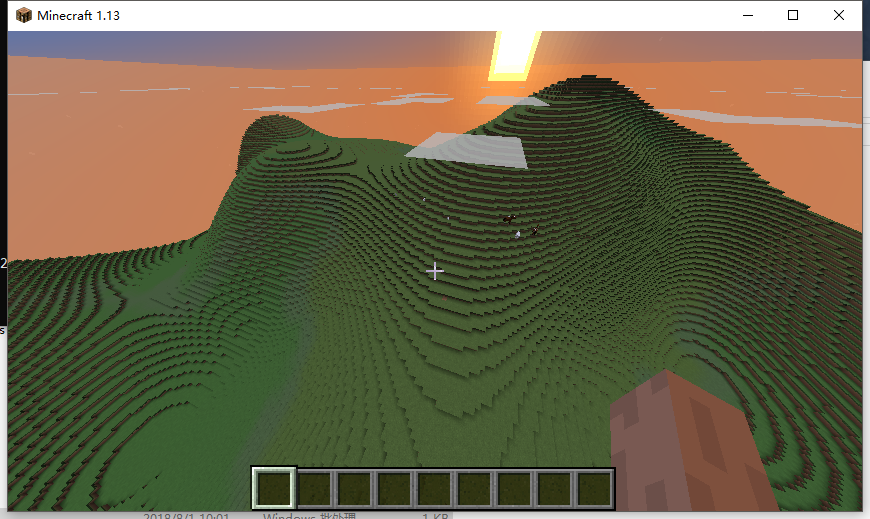### 加点东西

``````@Override
public List<BlockPopulator> getDefaultPopulators(World world) {
return ImmutableList.of(new TreePopulator());
}

private static class TreePopulator extends BlockPopulator {

@Override
public void populate(World world, Random random, Chunk chunk) {
// 假设只有 1/4 的区块生成树
if (random.nextInt(4) < 1) {
// 假设每个区块生成 1-3 颗树
int amount = random.nextInt(3) + 1;
for (int i = 0; i < amount; i++) {
// 随机生成树的坐标
int x = random.nextInt(16);
int z = random.nextInt(16);
int y = 255;
// 找到最高的方块来生成树
while (chunk.getBlock(x, y, z).getType() == Material.AIR) y--;
// 生成树
world.generateTree(chunk.getBlock(x, y, z).getLocation(),
// 搞点有趣的，我们随机选择不同的树生成
TreeType.values()[random.nextInt(TreeType.values().length)]);
}
}
}
}``````

（最终还是没有蘑菇树，果然还是不行呢）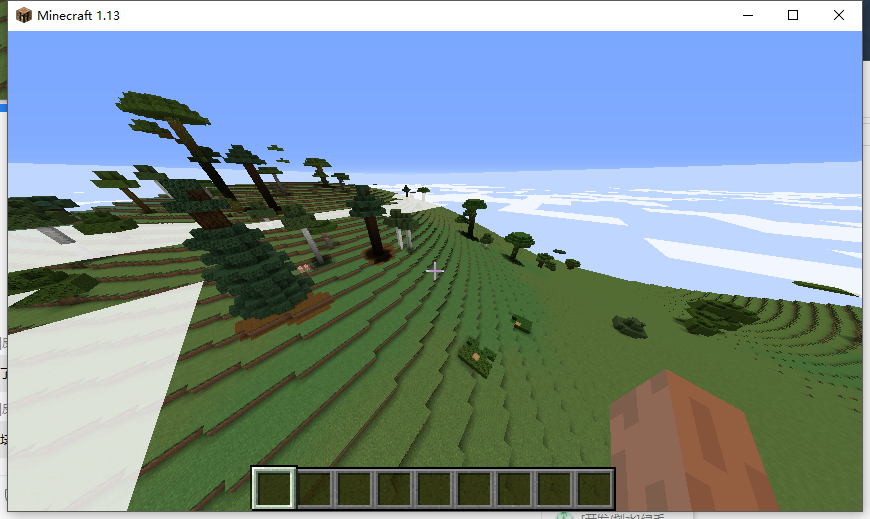``````@Override
public List<BlockPopulator> getDefaultPopulators(World world) {
return ImmutableList.of(new TreePopulator(), new DiamondPopulator());
}

private static class DiamondPopulator extends BlockPopulator {

@Override
public void populate(World world, Random random, Chunk chunk) {
// 假设每个区块只有一个钻石矿
// 钻石矿脉随机生成在高度 16 以下
int x = random.nextInt(16);
// 不要生成在基岩上
int y = random.nextInt(15) + 1;
int z = random.nextInt(16);
// 继续生成的几率
while (random.nextDouble() < 0.8D) {
// 只替换岩石
if (chunk.getBlock(x, y, z).getType() == Material.STONE) {
chunk.getBlock(x, y, z).setType(Material.DIAMOND_ORE);
}
// 向某个方向随机继续生成
switch (random.nextInt(6)) {
case 0: x++; break;
case 1: y++; break;
case 2: z++; break;
case 3: x--; break;
// 不要生成到基岩下面去了
case 4: y = Math.max(y-1, 0); break;
default: z--; break;
}
}
}
}``````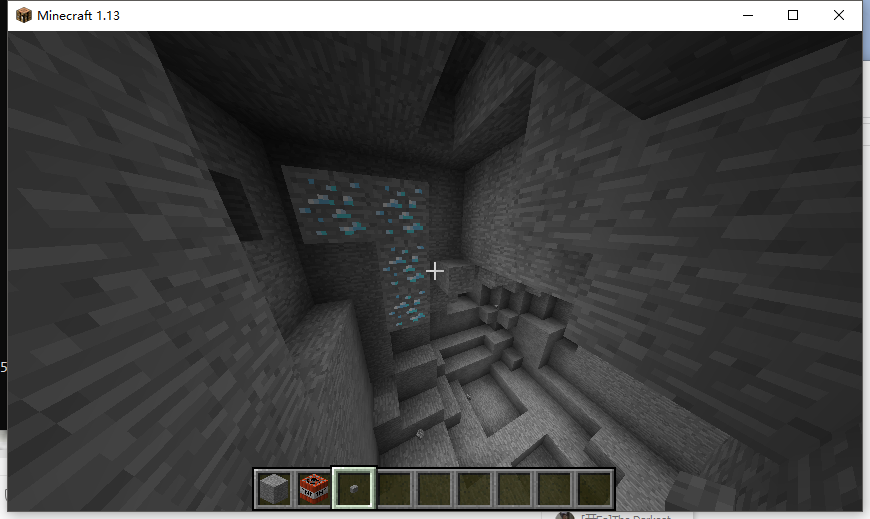## 玩转 NoiseGenerator / OctaveGenerator

### 噪声函数？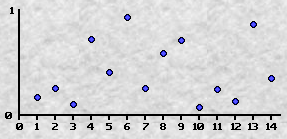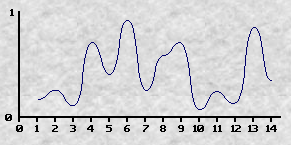### 函数的一些特性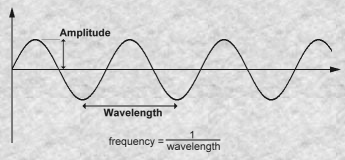• amplitude：振幅，为波的高度
• wavelength：波长，为每个峰之间的距离
• frequency：频率，为 1/波长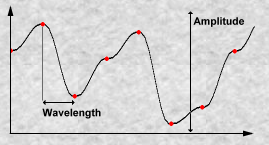• 每个红点代表函数的随机值
• 振幅为函数可能取得的最大值和最小值的差值
• 波长为每个红点之间的距离
• 频率仍然为 1/波长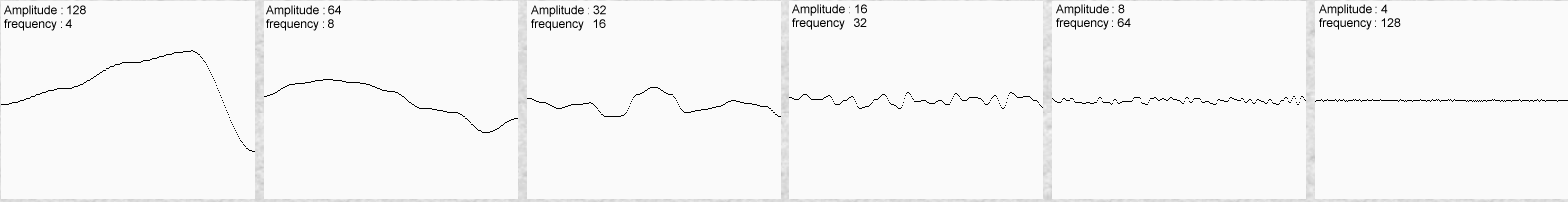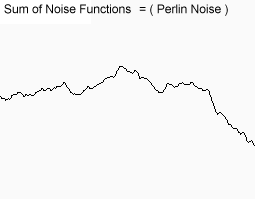### 数学表达式？

``f(x) = 1*noise(x) + 0.5*noise(2x) + 0.25*noise(4x) + 0.125*noise(8x) + 0.0625*noise(16x)``

### 代码？

``````/**
* 假设此函数返回 0-1 之间的随机数，并且满足噪声函数的相关定义
*
* @param x 参数
* @return 0-1 的随机数
*/
static double noise(double x) {
return 0;
}

/**
* 噪声函数
*
* @param x    参数
* @param freq 频率
* @param amp  振幅
* @return 函数值
*/
static double f(double x, double freq, double amp) {
return amp * noise(x * freq);
}

static double f(double x) {
return f(x, 1, 1) + f(x, 2, 0.5) + f(x, 4, 0.25) + f(x, 8, 0.125);
}``````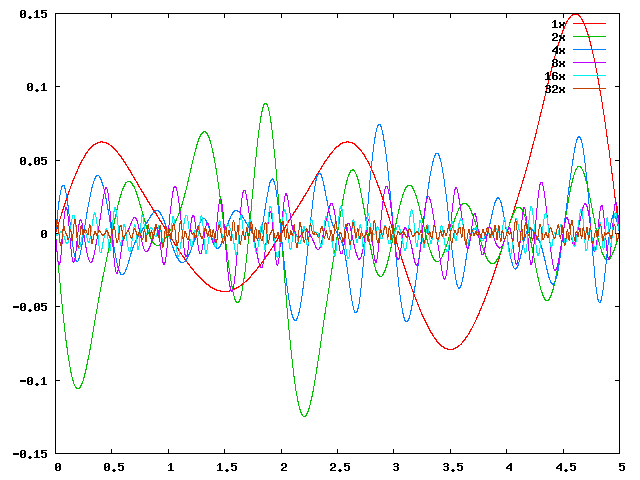``````static double f(double x, double freq, double amp, int octaves, boolean normalized) {
double result = 0.0D;
double a = 1.0D;
double f = 1.0D;
double max = 0.0D;

for (int i = 0; i < octaves; ++i) {
result += f(x, f, a);
max += amp;
f *= freq;
a *= amp;
}

if (normalized) {
result /= max;
}

return result;
}``````

### NoiseGenerator 与 OctaveGenerator

org.bukkit.util.noise 包内有 4 个类，用于提供生成噪声函数的方法。

``````static double bukkitNoise(double x, double freq, double amp, int octaves, boolean normalized) {
SimplexNoiseGenerator generator = new SimplexNoiseGenerator(new Random());
return generator.noise(x, octaves, freq, amp, normalized);
}

static double bukkitOctave(double x, double freq, double amp, int octaves, boolean normalized) {
SimplexOctaveGenerator generator = new SimplexOctaveGenerator(new Random(), octaves);
return generator.noise(x, freq, amp, normalized);
}``````

• Bukkit 的噪声函数类返回 -1 到 1 的值，与上文的 0 到 1 不同，自行处理即可
• Bukkit 的噪声函数类默认频率很大，所以需要使用小频率（OctaveGenerator 的 setScale(0.005)）
• 对于给定的相同种子，调用同一个点的函数总返回相同值

Perlin 是初代噪声函数，Simplex 基于 Perlin 优化，得到的图像更好看，在高维度的速度也更快。

## 噪声函数使用技巧

### Math.pow``````n1 = new PerlinNoiseGenerator(random);
double e = n1.noise(x * 0.01F, z * 0.01F, 6, 2.0F, 0.5F);
e = Math.pow(e, 2.5);
elevation[x][z] = (int) (64 + e * 64F);``````### Math.abs

``````function ridgenoise(x, z) {  return 2 * (0.5 - abs(0.5 - noise(x, z))); }
e0 =  1 * ridgenoise(1 * x, 1 * z);
e1 =  0.5 * ridgenoise(2 * x, 2 * z) * e0;
e2 = 0.25 * ridgenoise(4 * x, 4 * z) * (e0+e1);
e = e0 + e1 + e2;
elevation[x][z] = Math.pow(e, 2.5);``````